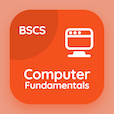Colleges Online Courses

Applied Physics Quizzes

Applied Physics Quiz PDF - Complete

# Vector Addition by Rectangular Components Multiple Choice Questions p. 2

Study Vector Addition by Rectangular Components multiple choice questions and answers, vector addition by rectangular components quiz answers PDF 2 to study Applied Physics course online. Vector and Equilibrium MCQ trivia questions, vector addition by rectangular components Multiple Choice Questions (MCQ) for online college degrees. "Vector Addition by Rectangular Components MCQ" PDF eBook: vector magnitude, applied physics: physical quantities, errors in measurements in physics, modern physics, vector addition by rectangular components test prep for two year degree programs.

"Direction of the resultant of two vectors is given by" MCQ PDF: cos-1(ax + by/ay + bx), cos-1(ay + by/ax + bx), tan-1(ay + by/ax + bx), and tan-1(ay - by/ax - bx) for online college classes. Learn vector and equilibrium questions and answers to improve problem solving skills for online college for teaching degree.

MCQ: Direction of the resultant of two vectors is given by

cos-1(Ay + By/Ax + Bx)
cos-1(Ax + By/Ay + Bx)
tan-1(Ay + By/Ax + Bx)
tan-1(Ay - By/Ax - Bx)

MCQ: Rest mass energy of an electron is

1.02 MeV
0.511KeV
0.511 MeV
2.02 MeV

MCQ: Errors that occur during measurement of the quantities are of

2 types
3 types
4 types
5 types

MCQ: Quantities that are used to describe the laws of physics are called

atomic quantities
nuclear quantities
mechanical quantities
physical quantities

MCQ: A vector having 'magnitude only' is called

scalar
resultant
unit vector
temperature

### More Quizzes from Applied Physics Course